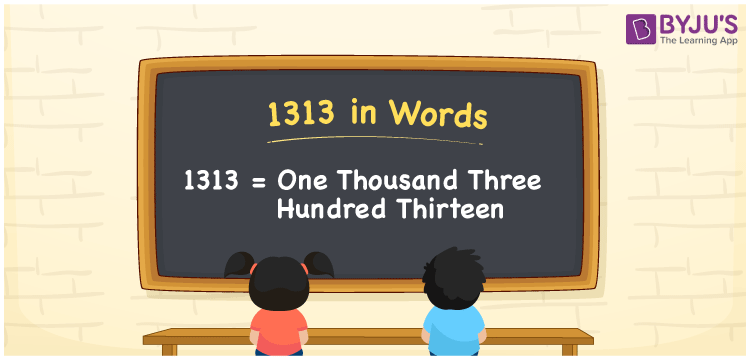# 1313 in Words

1313 in words is written as One thousand three hundred thirteen. In both the International System of Numerals and the Indian System of Numerals, 1313 is written as One thousand three hundred thirteen. The number 1313 is a Cardinal Number as it represents some quantity. For example, “1313 tickets for the concert are sold”.

 1313 in Words One thousand three hundred thirteen One thousand three hundred thirteen in Numerical Form 1313

## 1313 in English Words

1313 in English words is read as “One thousand three hundred thirteen”.## How to Write 1313 in Words?

To write 1313 in words, we shall use the place value chart. In the place value chart, put 1 in the thousands, 3 in the hundreds, 1 in the tens, and 3 in the ones, respectively. Let us make a place value chart to write the number 1313 in words.

 Thousands Hundreds Tens Ones 1 3 1 3

Thus, we can write the expanded form as

1 × Thousand + 3 × Hundred + 1 × Ten + 3 × One

= 1 × 1000 + 3 × 100 + 1 × 10 + 3 × 1

= 1000 + 300 + 10 + 3

= 1313

= One thousand three hundred thirteen.

1313 is a natural number which is the successor of 1312 and the predecessor of 1314.

1313 in words – One thousand three hundred thirteen

• Is 1313 an odd number? – Yes
• Is 1313 an even number? – No
• Is 1313 a perfect square number? – No
• Is 1313 a perfect cube number? – No
• Is 1313 a prime number? – No
• Is 1313 a composite number? – Yes

## Frequently Asked Questions on 1313 in Words

Q1

### How to write 1313 in words?

1313 in words is written as One thousand three hundred thirteen.
Q2

### How to write 1313 in the International and Indian System of Numerals?

In both, the system of numerals, 1313 in words, is written as One thousand three hundred thirteen.
Q3

### How to write 1313 in a place value chart?

In the place value chart, write 1 in the thousands, 3 in the hundreds, 1 in the tens, and 3 in the ones, respectively.
Test your Knowledge on 1313 in Words